#### FunctionalD

##### Description

FunctionalD[expr, {QuantumField[name, {mu}, {a}][p], ...}] calculates the functional derivative of expr with respect to the field list (with incoming momenta p, etc.) and does the fourier transform. FunctionalD[expr, {QuantumField[name, {mu},{a}], ...}] calculates the functional derivate and does partial integration but omits the x-space delta functions.

FunctionalD is a low level function used in FeynRule.

##### Examples

Instead of the usual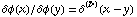the arguments and the δ function are omitted, i.e., for the program for simplicity: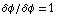``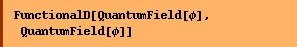``
`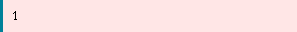`
``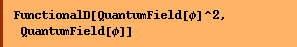``
`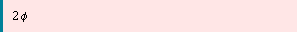`

Instead of the usual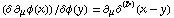the arguments are omitted, and the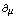operator is specified by default to be an integration by parts operator, i.e., the right hand side will be just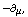or, more precisely (by default)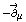.

``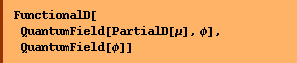``
`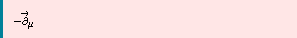`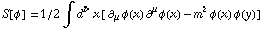``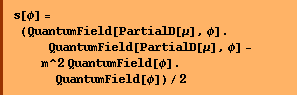``
`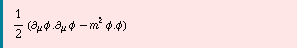`
``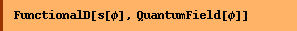``
`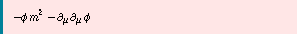`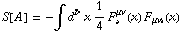First approach

``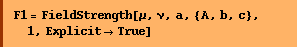``
`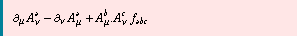`
``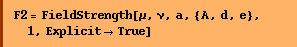``
`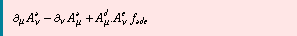`
``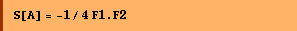``
`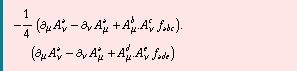`

In order to derive the equation of motion the functional derivative of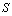with respect to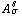has to be set to zero. Bearing in mind that for FeynCalc we have to be precise as to where which operators (coming from the substitution of the derivative of the δ function) act:, act with the functional derivative operator on the first field strength: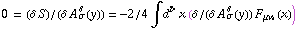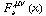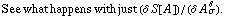``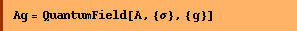``
`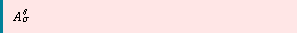`
``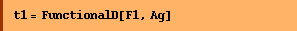``
`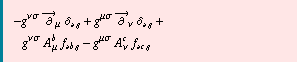`

In order to minimize the number of dummy indices, replace b -> c.

``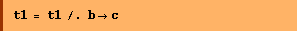``
`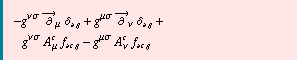`

Instead of inserting the definition for the second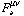, introduce a QuantumField object with antisymmetry built into the Lorentz indices:

``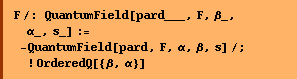``
``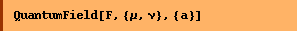``
`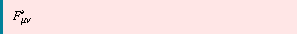`
``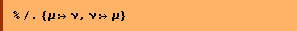``
`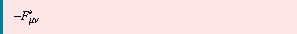`
``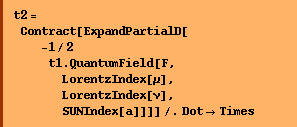``
`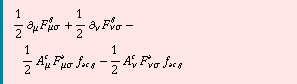`
``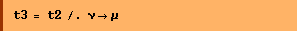``
`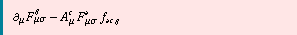`
``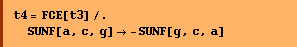``
`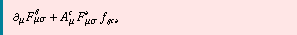`

Since the variational derivative vanishes t4 implies that 0 =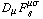.

Second approach

It is of course also possible to do the functional deriviate on the S[A] with both field strength tensors inserted.

``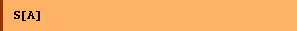``
``
``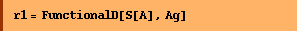``
`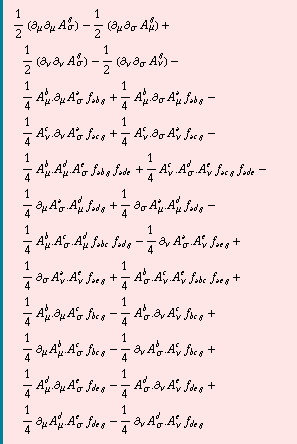`

This is just funcional derivatves and partial integration and simple contraction of indices. No attempt is made to rename dummy indices (since this is difficult in general ...).

With a general replacement rule only valid for commuting fields the color indices can be canonicalized a bit more. The idea is to use the commutative properties of the vector fields, and canonicalize the color indices by a trick. This function will work on any commuting product of fields.

``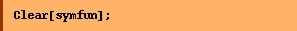``
``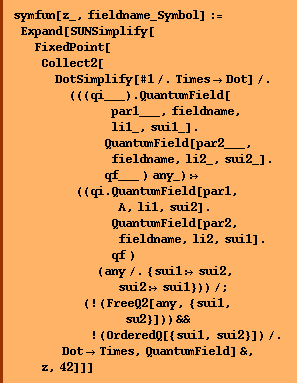``
``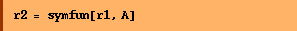``
`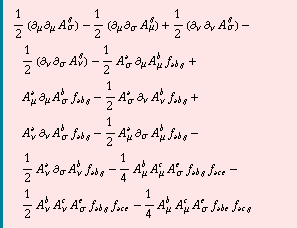`
``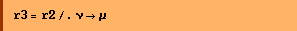``
`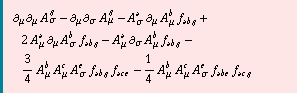`

Inspection reveals that still terms are the same. Gather the terms with two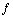's:

``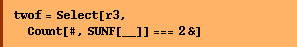``
`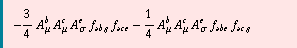`
``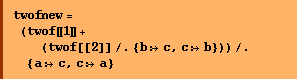``
`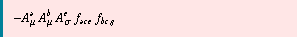`
``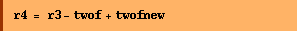``
`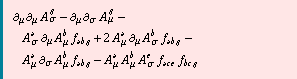`

Check that this is now indeed the same as the t4 result from the first attempt.

``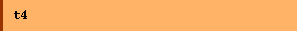``
``
``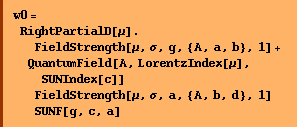``
`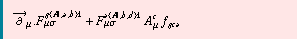`
``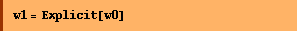``
`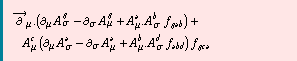`
``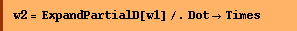``
`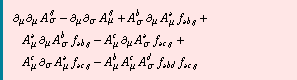`
``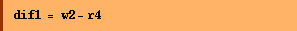``
`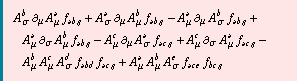`
``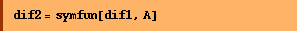``
`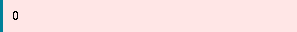`

quod erat demonstrandum.

``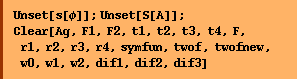``
##### Examples of funcional differentiation as used in FeynRule

This is a part of the QCD Lagrangian.

``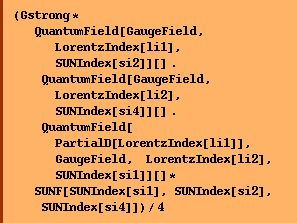``
`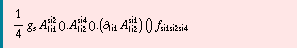`
``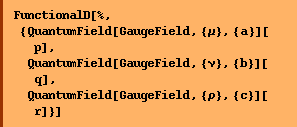``
`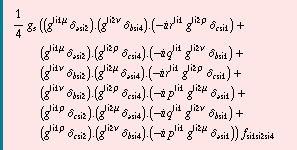`
``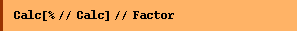``
`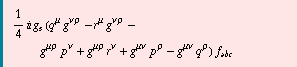`

Converted from the Mathematica notebook FunctionalD.nb